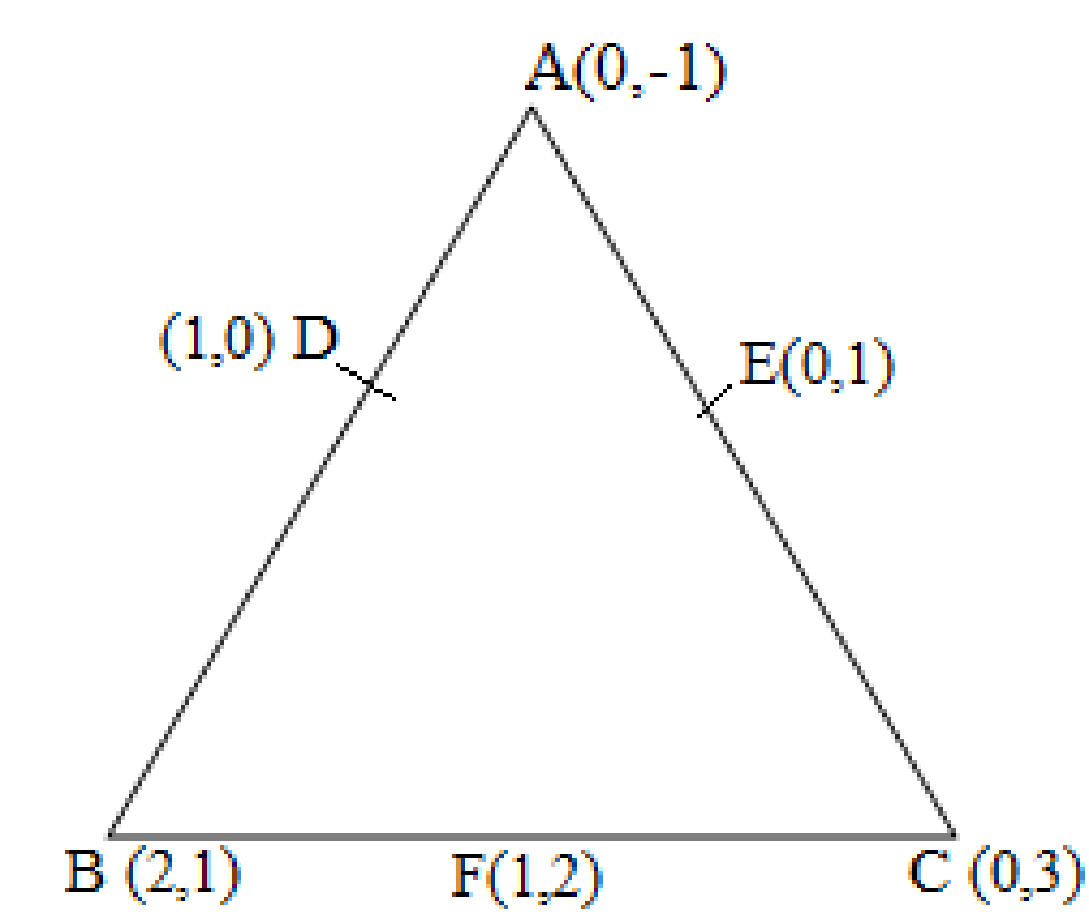Newbie

# Find the area of the triangle formed by joining the mid-points of the sides of the triangle whose vertices are (0, -1), (2, 1) and (0, 3). Find the ratio of this area to the area of the given triangle. Q 3

• 0

Coordinate geometry best and easy way to tackle the solution of exercise 7.3 can you show me the best solution.Find the area of the triangle formed by joining the mid-points of the sides of the triangle whose vertices are (0, -1), (2, 1) and (0, 3). Find the ratio of this area to the area of the given triangle.

Share

1. Let the vertices of the triangle be A (0, -1), B (2, 1), C (0, 3).

Let D, E, F be the mid-points of the sides of this triangle.

Coordinates of D, E, and F are given by

D = (0+2/2, -1+1/2 ) = (1, 0)

E = ( 0+0/2, -1+3/2 ) = (0, 1)

F = ( 0+2/2, 3+1/2 ) = (1, 2)Area of a triangle = 1/2 × [x1(y2 – y3) + x2(y3 – y1) + x3(y1 – y2)]

Area of ΔDEF = 1/2 {1(2-1) + 1(1-0) + 0(0-2)} = 1/2 (1+1) = 1

Area of ΔDEF is 1 square units

Area of ΔABC = 1/2 [0(1-3) + 2{3-(-1)} + 0(-1-1)] = 1/2 {8} = 4

Area of ΔABC is 4 square units

Therefore, the required ratio is 1:4.

• 0